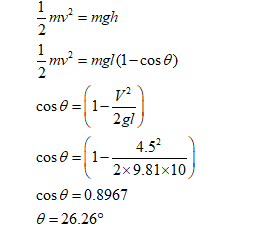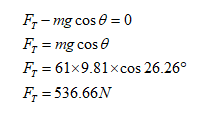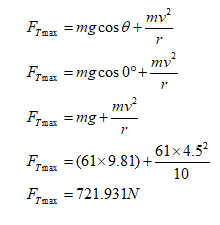# Problem 8.913 of 19Constants | Periodic TableA 61 kg student runs at 4.5 m/s , grabs a hangingrope, and swings out over a lake. He releases therope when his velocity is zero(Figure 1) .What is the angle e when he releases the rope?Express your answer using two significant figures.nν ΑΣφFigure< 1 of 1 >SubmitRequest AnswerPart B10.0 mWhat is the tension in the rope just before he releases it?Express your answer using two significant figures.Pearson Problem 8.913 of 19Constants | Periodic TableA 61 kg student runs at 4.5 m/s , grabs a hangingrope, and swings out over a lake. He releases therope when his velocity is zero(Figure 1)Part CWhat is the maximum tension in the rope?Figure< 1 of 1Express your answer using two significant figures.Hν ΑΣφFT =10.0 mSubmitRequest AnswerProvide FeedhackNext >

Question
6 views

check_circle

Step 1

Given:-

mass(m)=61 kg

velocity(v)=4.5 m/s

Lenght (L)=10m

Find.

A) what is the angle. when releases the rope

By conservation of energy.B) What is the tension in the rope just before he releases.

using Newton's second law for the radial.Step 2

C)What is the maximum tension in the role

from, Newton's second law...

### Want to see the full answer?

See Solution

#### Want to see this answer and more?

Solutions are written by subject experts who are available 24/7. Questions are typically answered within 1 hour.*

See Solution
*Response times may vary by subject and question.
Tagged in